# Lesson 21 AC Power and Power Triangle 1

• Slides: 17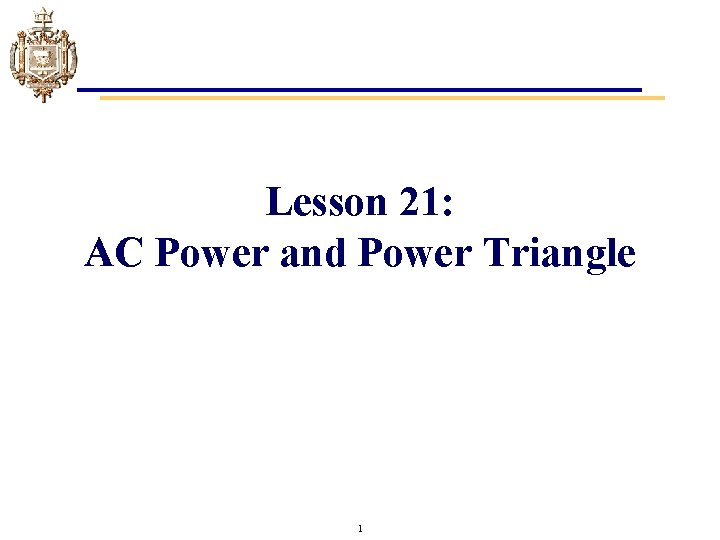Lesson 21: AC Power and Power Triangle 1Learning Objectives • Define real (active) power, reactive power, average, and apparent power. • Calculate the real, reactive, and apparent power in AC series parallel networks. • Graph the real and reactive power of purely resistive, inductive, or capacitive loads in AC series parallel networks as a function of time. • Determine when power is dissipated, stored, or released in purely resistive, inductive, or capacitive loads in AC series parallel networks. • Use the power triangle determine relationships between real, reactive and apparent power. 2AC Power • AC Impedance (Z) is a complex quantity made up of real resistance (R) and imaginary reactance (X): • AC Apparent Power (S) is a complex quantity made up of real active (P) power and imaginary reactive (Q) power: 3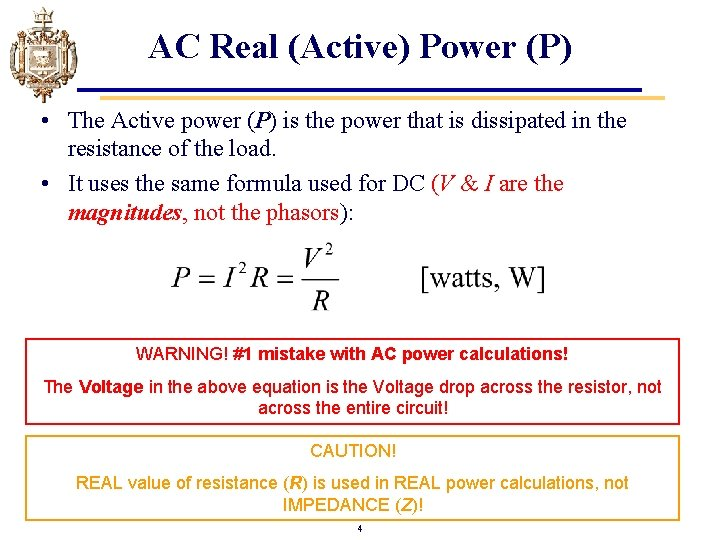AC Real (Active) Power (P) • The Active power (P) is the power that is dissipated in the resistance of the load. • It uses the same formula used for DC (V & I are the magnitudes, not the phasors): WARNING! #1 mistake with AC power calculations! The Voltage in the above equation is the Voltage drop across the resistor, not across the entire circuit! CAUTION! REAL value of resistance (R) is used in REAL power calculations, not IMPEDANCE (Z)! 4AC Imaginary (Reactive) Power (Q) • The reactive power (Q) is the power that is exchanged between reactive components (inductors and capacitors). • The formulas look similar to those used by the active power, but use reactance instead of resistances. WARNING! #1 mistake with AC power calculations! The Voltage in the above equation is the Voltage drop across the reactance, not across the entire circuit! • Units: Volts-Amps-Reactive (VAR) • Q is negative for a capacitor by convention and positive for inductor. − Just like X is negative for a capacitor! (-j. XC) 5AC Apparent Power (S) • The Apparent Power (S) is the power that is “appears” to flow to the load. • The magnitude of apparent power can be calculated using similar formulas to those for active or reactive power: • Units: Volts-Amps (VA) • V & I are the magnitudes, not the phasors. 6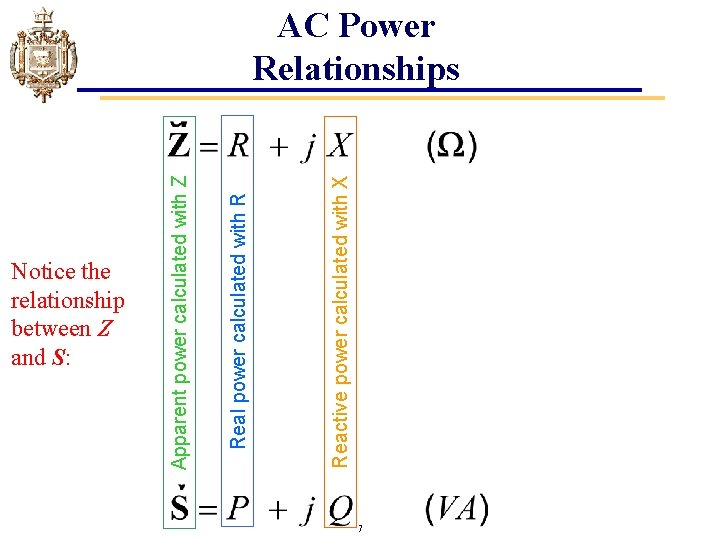Notice the relationship between Z and S: Reactive power calculated with X Real power calculated with R Apparent power calculated with Z AC Power Relationships 7Power Triangle • The power triangle graphically shows the relationship between real (P), reactive (Q) and apparent power (S). 8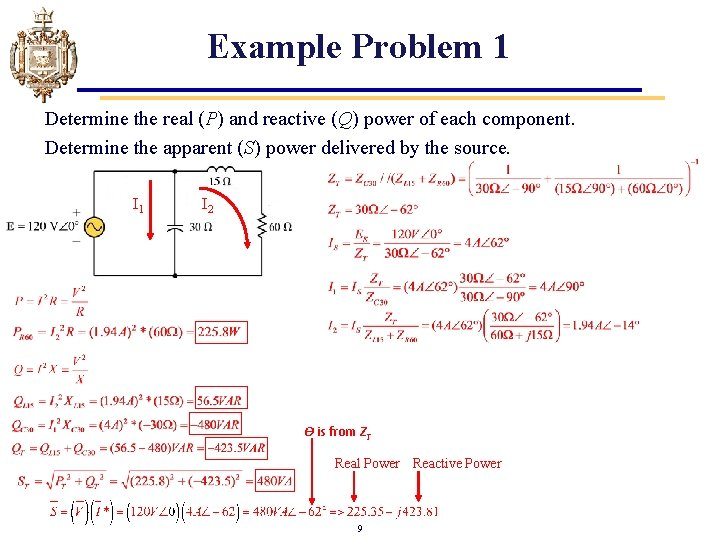Example Problem 1 Determine the real (P) and reactive (Q) power of each component. Determine the apparent (S) power delivered by the source. I 1 I 2 Ѳ is from ZT Real Power Reactive Power 9Real and Reactive Power • The power triangle also shows that we can find real (P) and reactive (Q) power. NOTE: The impedance angle and the “power factor angle” are the same value! 10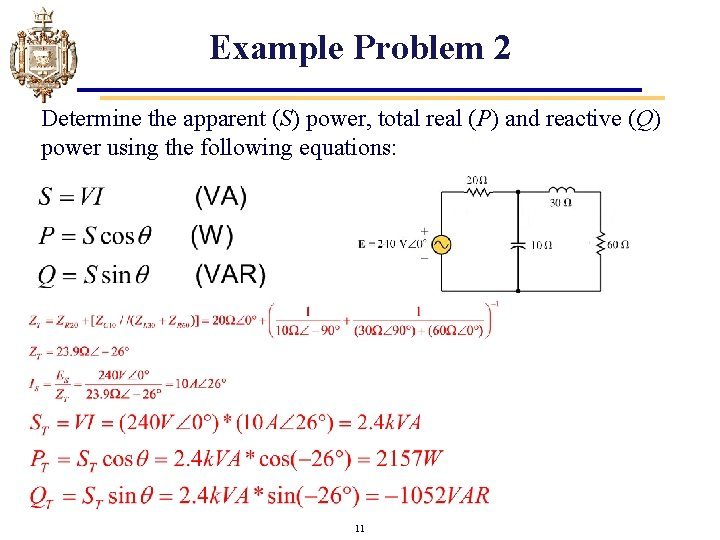Example Problem 2 Determine the apparent (S) power, total real (P) and reactive (Q) power using the following equations: 11Total Power in AC Circuits • The total power real (PT) and reactive power (QT) is simply the sum of the real and reactive power for each individual circuit elements. • How elements are connected does not matter for computation of total power. 12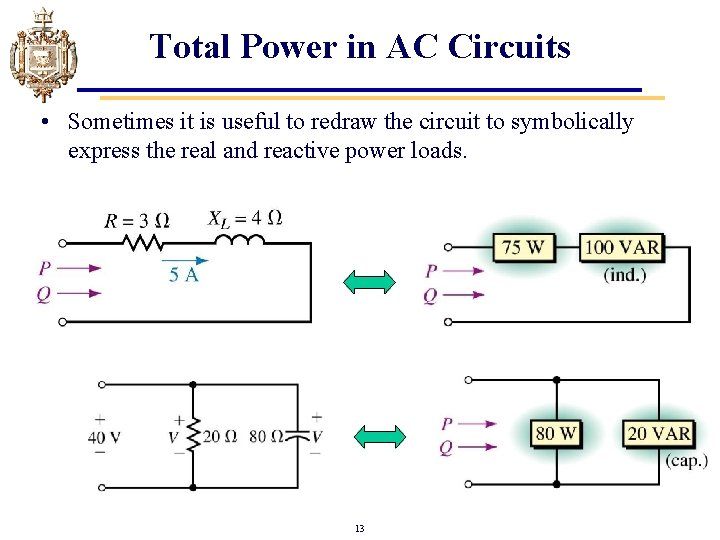Total Power in AC Circuits • Sometimes it is useful to redraw the circuit to symbolically express the real and reactive power loads. 13Example Problem 3 a. Determine the unknown real (P 2) and reactive powers (Q 3) in the circuit below. b. Determine total apparent power. c. Draw the power triangle. d. Is the unknown element in Load #3 an inductor or capacitor? Rearrange to find P 2: Like wise, for Q 3; rearrange to find Q 3: d) Because Q 3 is negative, it is capacitive 14 QT = -1. 1 k. VAR c) Power Triangle PT = 2. k. W = 3 S T 2 k. 10 VAExample Problem 4 a. b. Determine the value of R, PT and QT Draw the power triangle and determine S. 15Use of Complex Numbers in Power Calculations • AC power can be calculated using complex equations. • Apparent Power can be represented as a complex number. • The resultant can be used to determine real and reactive power by changing it to rectangular form. NOTE! The complex conjugate of Current is used to make the power angle the same as the impedance angle! 16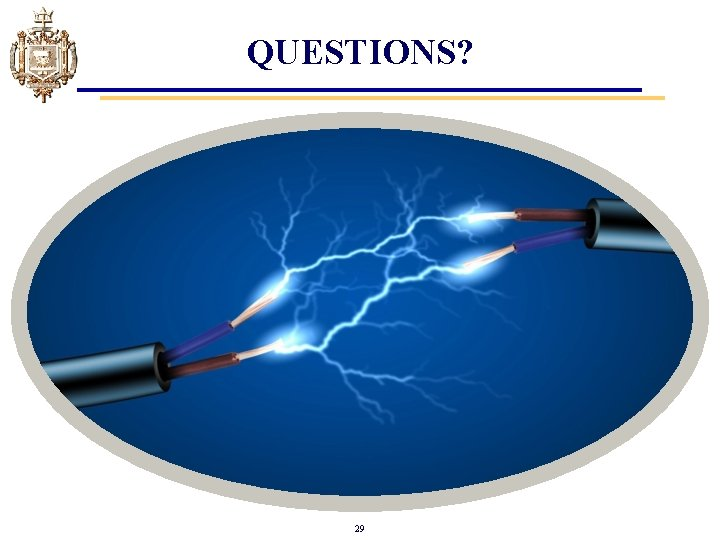QUESTIONS? 29